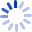# Regression Diagnostics: An Introduction

by

Regression diagnostics are methods for determining whether a regression model that has been fit to data adequately represents the structure of the data. For example, if the model assumes a linear (straight-line) relationship between the response and an explanatory variable, is the assumption of linearity warranted? Regression diagnostics not only reveal deficiencies in a regression model that has been fit to data but in many instances may suggest how the model can be improved. The Second Edition of this bestselling volume ...

##### Cash for Textbooks